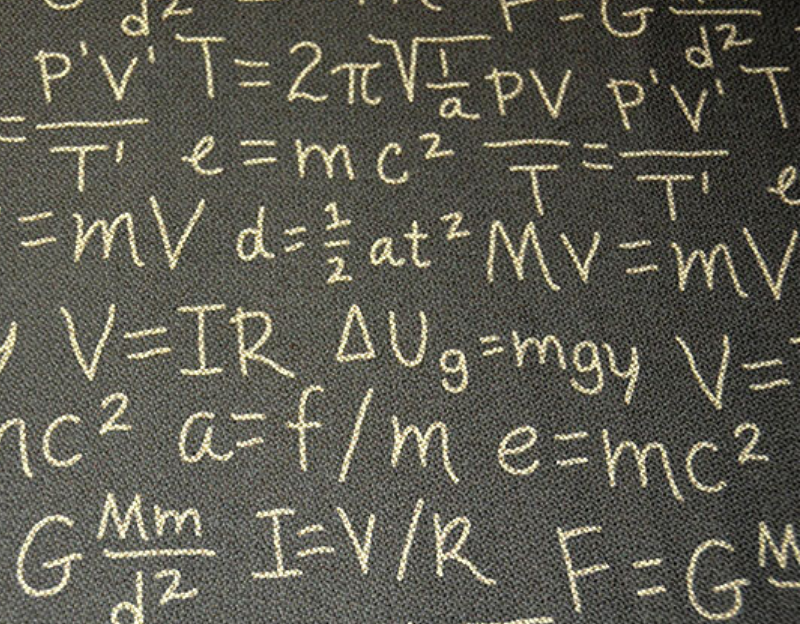# An Overview of The Relationship Between Math and Physics

Mar 02, 2020The relationship between mathematics and physics has always been a subject of study for philosophers, physicists, and mathematicians. This relationship in one word is often described to be as “intimate”.

Mathematics is always considered to be an “essential tool for Physics.” Physics, on the other hand, is also considered to be a “rich source of inspiration and insight in mathematics.” So we can already see from the two statements that those two subjects are definitely inter-related to each other. Let’s see to it in more detail.

## From history

Aristotle Physics has a topic that is based on the ways through which a study carried out by mathematicians differs considerably from the one done by a physicist.

From the 17th century, much significant advancement in mathematics seems to be motivated by the study of physics.

A simple example can be calculus. The creation of calculus and its development were strongly related to physics. Newton also regarded geometry to be an active branch of mechanics and the way we see it now; we are sure that he was right at that time.

As time progressed, more sophisticated mathematics came into existence and more and more of them started using physics. The current situation at that moment is that the mathematical knowledge utilized in physics is getting increasingly sophisticated.

## Mathematics is used to comprehend the relationship between objects

Mathematics is the language of devising the empirical laws of nature.

The main motto of physics is to explain the relationship between various measurements of physical objects. A large portion of mathematics is devoted to comprehending and defining the relationships between objects. Therefore, the application of mathematics in physics is undeniable if you consider this aspect common in both the subjects.

## Modern examples

Almost all topics of physics included in the school curriculum incorporate a whole lot of mathematics in their underlying principles. These topics are:

Measurements: Ranging from the measurement of the length of an object using Vernier Calipers to the measurement of the speed of light, mathematics is applicable in all such cases and all the ones in between.

Kinematics: This is the branch of physics (or more precisely we should say mechanics) that’s concerned with the motion of objects without referring to the forces that cause the motion. Kinematics is increasingly based on mathematics. Each and every calculation in the subject can easily justify this claim.

Newton’s laws of motion: All the three laws of motion incorporate a huge deal of mathematics to come to those conclusions.

Work, energy and power

Motion of System of Particles and Rigid Body

Gravitation

Oscillations and Waves

There are countless other physics topics that are inherently based on mathematics. You’ll definitely realize the connection yourself when you learn about a specific physics principle in exquisite detail.

So you have definitely seen that physics and mathematics do go hand in hand. But if you still have some doubts for which you are asking yourself this question as to why mathematics is useful in physics, a simple counter-question from our end should clear that up. Asking “why is math so useful in physics?” is almost similar to asking “why is math useful in measuring a kilo of apples?” With that, we’ll bring this article to an end. Hope to see you back soon. Ciao!